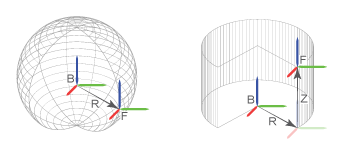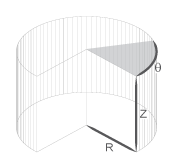## Translational Measurements

### Translation Sensing Overview

You can measure frame translation in different coordinate systems. These include Cartesian, cylindrical, and spherical systems. The different coordinate systems are available through the Transform Sensor block and, to a limited extent, through the Joint blocks. The choice of coordinate system depends on the model. Select the coordinate system that is most convenient for your application.

### Measuring Translation

Translation is a relative quantity. The translation of one frame is meaningful only with respect to another frame. As such, blocks with translation sensing capability require two frames to make a measurement: measured and reference frames. In these blocks, the follower frame port identifies the measured frame; the base frame port identifies the reference frame of the measurement.

Some measurements are common to multiple coordinate systems. One example is the Z-coordinate, which exists in both Cartesian and cylindrical systems. In the Transform Sensor dialog box, coordinates that make up more than one coordinate system appear only once. Selecting Z outputs translation along the Z-axis in both Cartesian and cylindrical coordinate systems.

Other measurements are different but share the same name. For example, radius is a coordinate in both spherical and cylindrical systems. The spherical radius is different from the cylindrical radius: the former is the distance between two frame origins; the latter is the distance between one frame origin and a frame Z-axis.To differentiate between the two radial coordinates, Simscape™ Multibody™ uses the following convention:

• Distance — Spherical radial coordinate

### Cartesian Measurements

The Cartesian coordinate system uses three linear coordinates—X, Y, and Z—corresponding to three mutually orthogonal axes. Cartesian translation measurements have units of distance, with meter being the default. You can use the PS-Simulink Converter block to select a different physical unit when interfacing with Simulink® blocks.#### Transform Sensor

You can select any of the Cartesian axes in the Transform Sensor for translation sensing. This is true even if translation is constrained along any of the Cartesian axes. Selecting the Cartesian axes exposes physical signal ports x, y, and z, respectively.

#### Joints

With joint blocks, you can sense translation along each prismatic primitive axis. Selecting a sensing parameter from a prismatic primitive menu exposes the corresponding physical signal port. For example, if you select Position from the Z Prismatic Primitive (Pz) of a Cartesian Joint block, the block exposes physical signal port z.

### Cylindrical Measurements

The cylindrical coordinate system uses one angular and two linear coordinates. The linear coordinates are the cylinder radius, R, and length, Z. The angular coordinate is the azimuth, ϕ, about the length axis. Linear coordinates have units of distance, with meter being the default. The angular coordinate has units of angle, with radian being the default. You can use the PS-Simulink Converter block to select a different physical unit when interfacing with Simulink blocks.#### Transform Sensor

Only the Transform Sensor block can sense frame translation in cylindrical coordinates. In the dialog box of this block, you can select one or more cylindrical coordinates to measure. The cylindrical coordinates are named Z, Radius, and Azimuth. Selecting the cylindrical coordinates exposes physical signal ports z, rad, and azm, respectively.

Note

Z belongs to both Cartesian and cylindrical systems.

### Spherical Measurements

The spherical coordinate system uses two angular coordinates and one linear coordinate. The linear coordinate is the spherical radius, R. The angular coordinates are the azimuth, ϕ, and inclination, θ. The linear coordinate has units of distance, with meter being the default. The angular coordinates have units of angle, with radian being the default. You can use the PS-Simulink Converter block to select a different physical unit when interfacing with Simulink blocks.#### Transform Sensor

Only the Transform Sensor block can sense frame translation in spherical coordinates. In the dialog box of this block, you can select one or more spherical coordinates to measure. The spherical coordinates are named Azimuth, Distance, and Inclination. Selecting the spherical coordinates exposes physical signal ports azm, dst, and inc, respectively.

Note

Azimuth belongs to both cylindrical and spherical systems. Distance is the spherical radius.